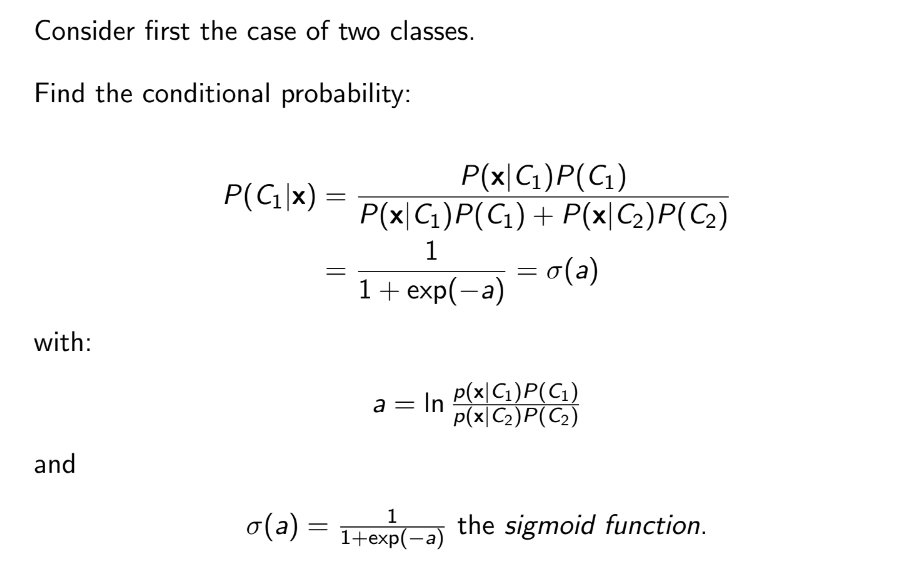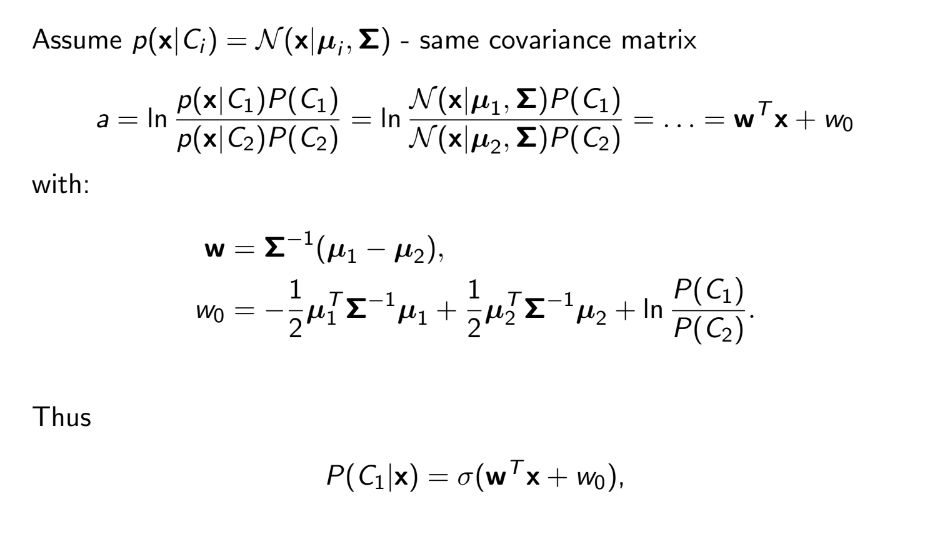# #StackBounty: #machine-learning #classification #probability #bayesian Problem understanding probabilistic generative models for classi…

### Bounty: 50

I am a student and I am studying machine learning. I am focusing on probabilistic generative models for classification and I am having some troubles understanding this topic.

In the slide of my professor it is written the following:which I don’t understand.

So far, I have understood that in the generative probailistic models, we ant to estimate $$P(C_i|x)$$, which is the probability of having class $$i$$ given a data $$x$$, using the likelihood and the Bayes theorem.

So, it starts by writing the Bayes rule, but the the slides says that we can write this as a sigmoid, but why?

If I have to try to give an answer to it, I would say because the sigmoid gives a number from $$0$$ to $$1$$, and so a probability, but it is just a guess I am doing.

Moreover, it continues by saying that we can use a gaussian distribution for $$P(x|C_i)$$, and so $$P(x|C_i)=N(mu ,sigma )$$, and so :